Anúncio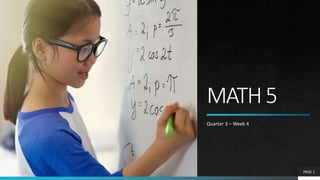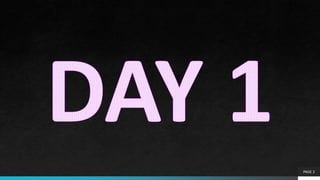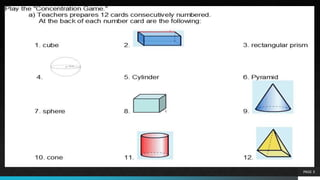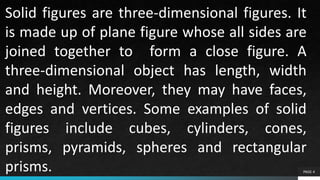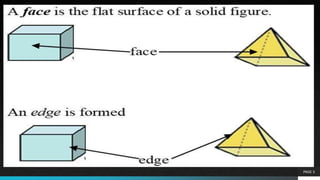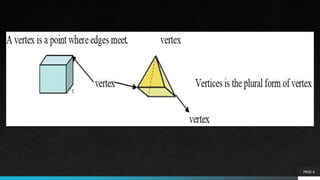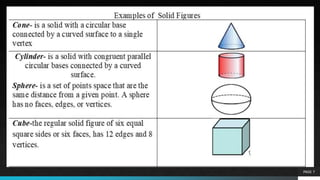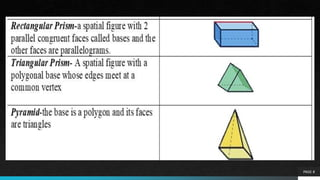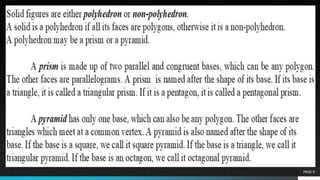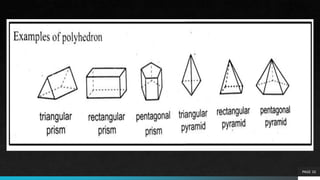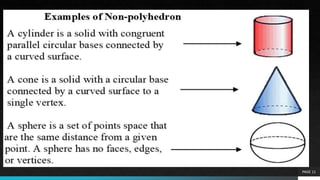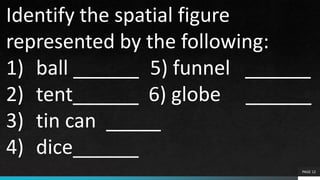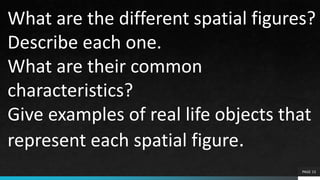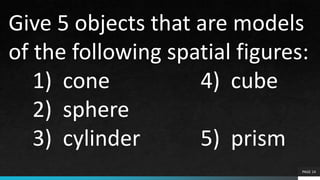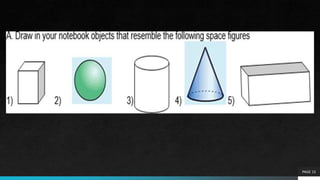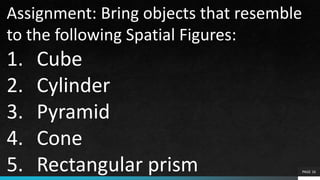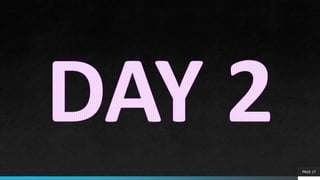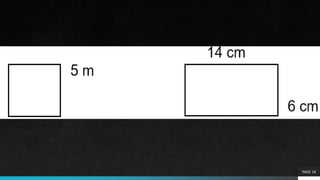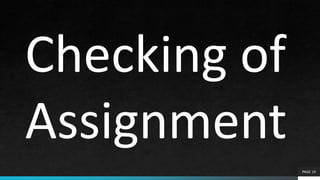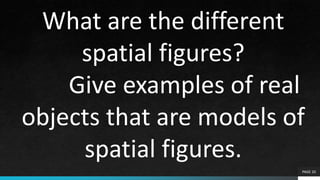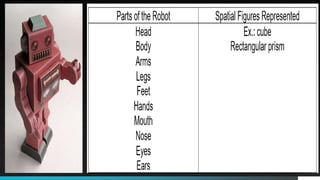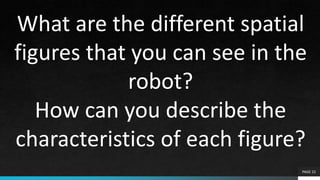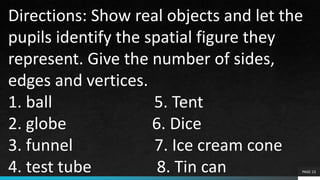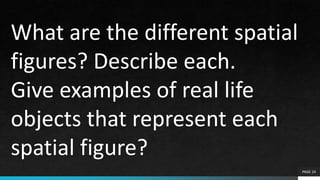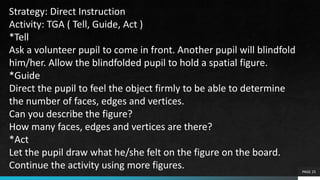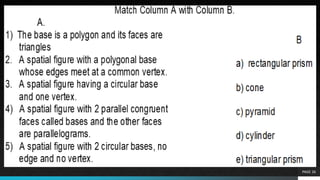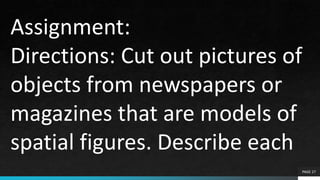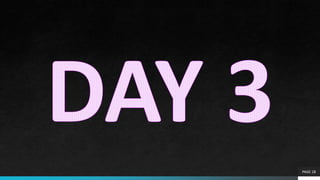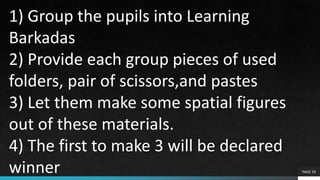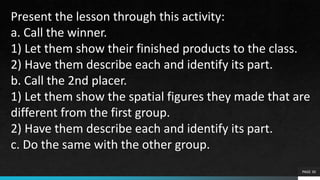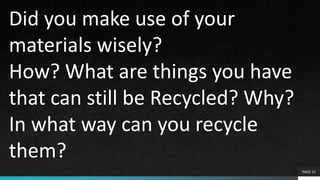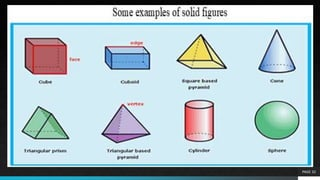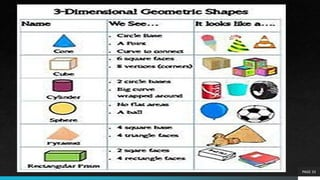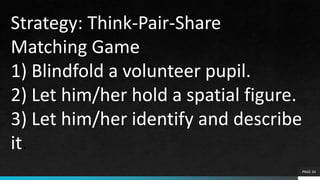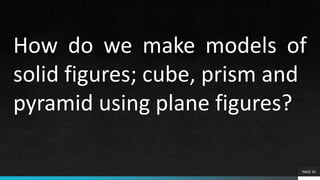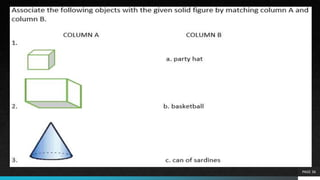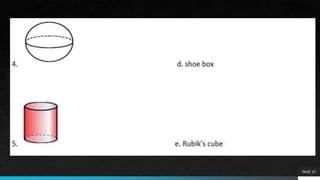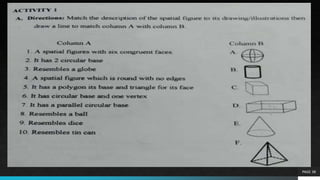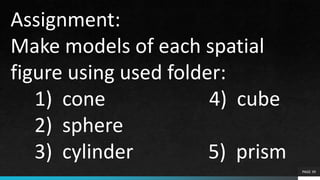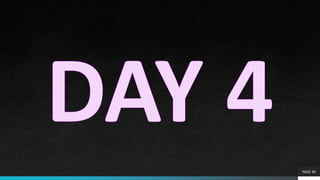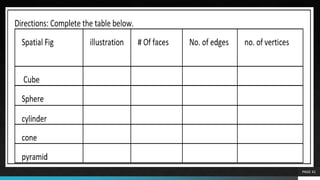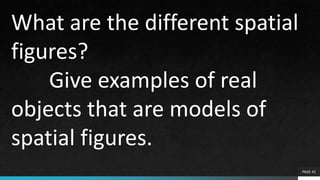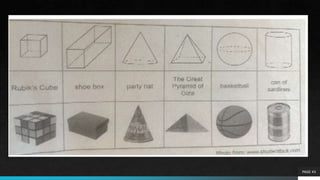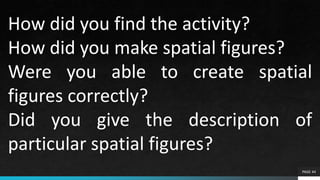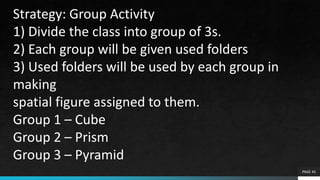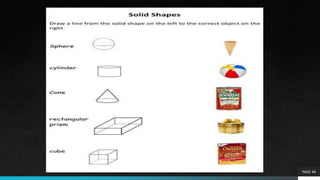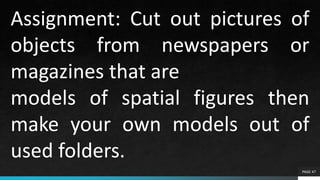1 de 47
Anúncio

### MATH 5.pptx

1. MATH5 Quarter 3 – Week 4 PAGE 1
2. PAGE 2
3. PAGE 3
4. PAGE 4 Solid figures are three-dimensional figures. It is made up of plane figure whose all sides are joined together to form a close figure. A three-dimensional object has length, width and height. Moreover, they may have faces, edges and vertices. Some examples of solid figures include cubes, cylinders, cones, prisms, pyramids, spheres and rectangular prisms.
5. PAGE 5
6. PAGE 6
7. PAGE 7
8. PAGE 8
9. PAGE 9
10. PAGE 10
11. PAGE 11
12. PAGE 12 Identify the spatial figure represented by the following: 1) ball ______ 5) funnel ______ 2) tent______ 6) globe ______ 3) tin can _____ 4) dice______
13. PAGE 13 What are the different spatial figures? Describe each one. What are their common characteristics? Give examples of real life objects that represent each spatial figure.
14. PAGE 14 Give 5 objects that are models of the following spatial figures: 1) cone 4) cube 2) sphere 3) cylinder 5) prism
15. PAGE 15
16. PAGE 16 Assignment: Bring objects that resemble to the following Spatial Figures: 1. Cube 2. Cylinder 3. Pyramid 4. Cone 5. Rectangular prism
17. PAGE 17
18. PAGE 18
19. PAGE 19 Checking of Assignment
20. PAGE 20 What are the different spatial figures? Give examples of real objects that are models of spatial figures.
21. PAGE 21
22. PAGE 22 What are the different spatial figures that you can see in the robot? How can you describe the characteristics of each figure?
23. PAGE 23 Directions: Show real objects and let the pupils identify the spatial figure they represent. Give the number of sides, edges and vertices. 1. ball 5. Tent 2. globe 6. Dice 3. funnel 7. Ice cream cone 4. test tube 8. Tin can
24. PAGE 24 What are the different spatial figures? Describe each. Give examples of real life objects that represent each spatial figure?
25. PAGE 25 Strategy: Direct Instruction Activity: TGA ( Tell, Guide, Act ) *Tell Ask a volunteer pupil to come in front. Another pupil will blindfold him/her. Allow the blindfolded pupil to hold a spatial figure. *Guide Direct the pupil to feel the object firmly to be able to determine the number of faces, edges and vertices. Can you describe the figure? How many faces, edges and vertices are there? *Act Let the pupil draw what he/she felt on the figure on the board. Continue the activity using more figures.
26. PAGE 26
27. PAGE 27 Assignment: Directions: Cut out pictures of objects from newspapers or magazines that are models of spatial figures. Describe each
28. PAGE 28
29. PAGE 29 1) Group the pupils into Learning Barkadas 2) Provide each group pieces of used folders, pair of scissors,and pastes 3) Let them make some spatial figures out of these materials. 4) The first to make 3 will be declared winner
30. PAGE 30 Present the lesson through this activity: a. Call the winner. 1) Let them show their finished products to the class. 2) Have them describe each and identify its part. b. Call the 2nd placer. 1) Let them show the spatial figures they made that are different from the first group. 2) Have them describe each and identify its part. c. Do the same with the other group.
31. PAGE 31 Did you make use of your materials wisely? How? What are things you have that can still be Recycled? Why? In what way can you recycle them?
32. PAGE 32
33. PAGE 33
34. PAGE 34 Strategy: Think-Pair-Share Matching Game 1) Blindfold a volunteer pupil. 2) Let him/her hold a spatial figure. 3) Let him/her identify and describe it.
35. PAGE 35 How do we make models of solid figures; cube, prism and pyramid using plane figures?
36. PAGE 36
37. PAGE 37
38. PAGE 38
39. PAGE 39 Assignment: Make models of each spatial figure using used folder: 1) cone 4) cube 2) sphere 3) cylinder 5) prism
40. PAGE 40
41. PAGE 41
42. PAGE 42 What are the different spatial figures? Give examples of real objects that are models of spatial figures.
43. PAGE 43
44. PAGE 44 How did you find the activity? How did you make spatial figures? Were you able to create spatial figures correctly? Did you give the description of particular spatial figures?
45. PAGE 45 Strategy: Group Activity 1) Divide the class into group of 3s. 2) Each group will be given used folders 3) Used folders will be used by each group in making spatial figure assigned to them. Group 1 – Cube Group 2 – Prism Group 3 – Pyramid
46. PAGE 46
47. PAGE 47 Assignment: Cut out pictures of objects from newspapers or magazines that are models of spatial figures then make your own models out of used folders.

### Notas do Editor

1. Show different spatial figures like the cone, cube, rectangular prism, cylinder, sphere and pyramid. Ask the pupils to name them. Let them give / count the number of edges, faces and vertices.
2. These figures are often seen in real life. Can you think of other objects that may represent the figures above?
Anúncio